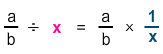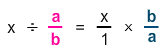6,457 Plays
Rate Quiz 0 stars
Quiz size:
Message preview:
Someone you know has shared quiz with you:

To play this quiz, click on the link below:

https://www.turtlediary.com/quiz/dividing-with-fractions-and-whole-numbers.html

Hope you have a good experience with this site and recommend to your friends too.

Login to rate activities and track progress.
Login to rate activities and track progress.
CCSS.Math.Content.5.NF.B.3

# Dividing With Fractions and Whole Numbers

Dividing a fraction by a whole number is same as multiplying by its reciprocal.For example: Let's find the quotient.

 5 7
÷
 4

Dividing a fraction by a whole number is same as multiplying by its reciprocal.

 5 7
÷
 4 1
=
 5 7
×
 1 4
 5 7
×
 1 4
=
 5 × 1 7 × 4
=
 5 28
So,
 5 7
÷   4  =
 5 28

Dividing a whole number by a fraction is same as multiplying by its reciprocal.For example: Let's find the quotient.

27  ÷
 5 2

Dividing a fraction by a whole number is same as multiplying by its reciprocal.

 27 1
÷
 5 2
=
 27 1
×
 2 5

 27 1
×
 2 5
=
 27 × 2 1 × 5
=
 54 5
=
 10 4 5

So,  27   ÷
 5 2
=
 10 4 5

ds

A B C D E F G H I J K L M N O P Q R S T U V W X Y Z